Part 1 | IFT World
IFT Notes for Level I CFA® Program
IFT Notes for Level I CFA® Program

# Part 1

## 1. Introduction

This learning module covers:

• How to value a derivative using a one-period binomial model
• The concept of risk-neutrality in derivatives pricing

## 2. Binomial Valuation

According to the law of one price, if the payoffs from any two assets at a given future date are identical, then the value of these two assets must also be identical today.

Forward commitments can be priced without making assumptions about the future price of the underlying asset. However, due to their asymmetric payoffs, the pricing of options requires a model to factor in the random price behavior of the underlying asset.

A commonly used model to determine the no-arbitrage value of an option is the binomial model.

## 3. The Binomial Model

The binomial model assumes that over a given time period, there are only two possible outcomes – the asset’s price will either go up to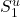or down to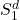.
Let q denote the probability of an upward price movement and 1 – q denote the probability of a downward price movement. With only two possible outcomes, the sum of these two probabilities must equal to 1.
To value options, knowing q is not required, only the valuesandare needed. The difference between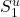andrepresents the spread of possible future price outcomes or the volatility of the underlying asset.
We can also define the upward and downward moves in terms of returns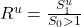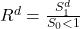Exhibit 1 from the curriculum, illustrates the binomial model.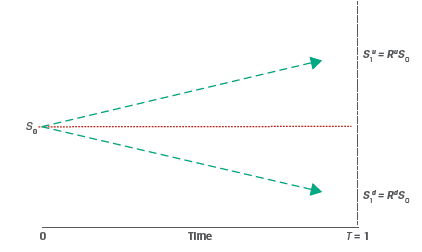## 4. Pricing a European Call Option

Consider a one-year European call option with an exercise price (X) of 100.

The underlying spot price (S0) is 80.

The two possible stock prices after 1 year are:= 60 and=110

We can compute the up and down returns as:
Ru = 110/80 = 1.375
Rd = 60/80 = 0.75

At t = 0, the call option value is c0.
At t = 1, the call option value is either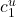(if the price goes up) or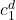(if the price goes down). We can compute the valuesandas:

Up move: The call option ends in-the-money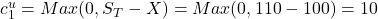Down move: The call option ends out-of-the-money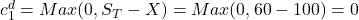To compute c0 we can use replication and no-arbitrage pricing. Assume that at t = 0 we sell the call option at a price of c0 and purchase h units of the underlying asset. V0 represents the initial investment in the portfolio, and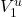and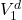represent the portfolio value if the underlying price moves up or down, respectively.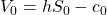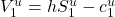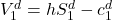We solve for h, such that the portfolio payoff in both the up and down scenarios are equal, i.e.: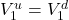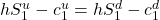Solving for h, we get: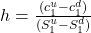This equation gives us the hedge ratio, or the proportion of the underlying that will offset the risk associated with an option.
The hedge ratio for our example is:
h=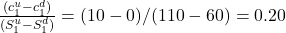i.e. for each call option unit sold, we must buy 0.2 units of the underlying asset.
The portfolio values at t=1 for the up and down scenarios are: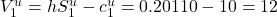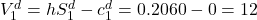As expected, the portfolio values are the same, which has two implications:
We can use either portfolio to value the derivative, and
The return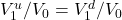must equal one plus the risk-free rate.

To prevent arbitrage, the portfolio value at t =1 should be discounted at the risk-free rate so that: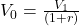For our example, V1 =12. Assume that the annual risk-free rate is 5%. Therefore,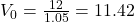Replacing V0 by the hedged portfolio we get: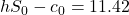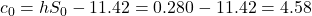The value of the call option at t = 0 is 4.58.

Example:

(This is based on Example 1 from the curriculum.)

Hightest Capital believes that a particular non-dividend-paying stock is currently trading at $50 and is considering the sale of a one-year European call option at an exercise price of$55.

If the stock price is expected to either go up or down by 20% over the next year, what price should Hightest expect to receive for the sold call option? Assume a risk-free rate of 5%.

Solution:

S0 = 50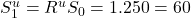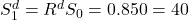The value of the call option at expiry is: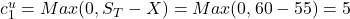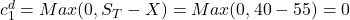The hedge ratio of the option is: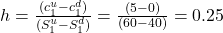The value of the hedged portfolio at maturity is: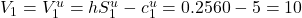The present value of the hedged position today is:

V0 = V1(1 + r)−1 = $10.00 × 1.05−1 = 9.52 The call option value is: c0 = h*S0 − V0 = 0.25 × 50.00 − 9.52 = 2.98 ## 5. Risk Neutrality As seen in the previous section, neither the actual (real-world) probabilities of underlying price increases or decreases nor the expected return of the underlying are required to price an option. Only the expected volatility i.e. the values S_1^u and S_1^d are required to price an option. Another method of calculating the value of a call option today, c0, is as the expected value of the option at expiration, c_1^u and c_1^d, discounted at the risk-free rate, r.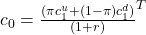In this equation π and (1-π) represent risk-neutral probabilities (rather than actual probabilities) of an up move and down move respectively. The risk-neutral probability can be calculated as: π=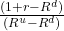Substituting the details from our earlier example: Ru = 1.375, Rd = 0.75,= 10,=0 and assuming an annual risk-free rate of 5%, we get: π=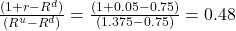We can compute the value of c0 as: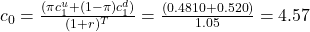This matches our result from the previous section. The risk-neutral probabilities used in this method are determined solely by the up and down gross returns, Ru and Rd, representing underlying asset volatility and the risk-free rate. The investor view on risk is not considered hence this method is referred to as risk-neutral pricing. Example: (This is based on Example 2 from the curriculum.) Using the same details as Example 1: “Hightest Capital believes that a particular non-dividend-paying stock is currently trading at$50 and is considering the sale of a one-year European call option at an exercise price of \$55.

If the stock price is expected to either go up or down by 20% over the next year, what price should Hightest expect to receive for the sold call option? Assume a risk-free rate of 5%.”

1. Compute the risk-neutral probability of an up move and down move.
2. Calculate the no-arbitrage price of the call option.

Solution to 1:

The risk neutral probability of an up move is:
π=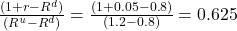The risk-neutral probability of a down move is therefore:
1 – π = 1 – 0.625 = 0.375.

Solution to 2: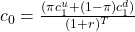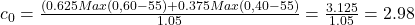LIVE WEEKEND CLASSES *For 2024 Exam candidates*
This is default text for notification bar